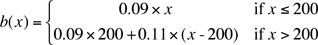I have two problems that have to be set up in an equation form and I don't know how to set them up in the equation form, the way my instructor wants me to, I hope you can help me! First Problem: Southeast Electric charges .09 cents per kilowatt-hour for the first 200 kWh.The company charges .11 cents per kilowatt-hour for all electrical usage in excess of 200 kWh. How many kilowatt-hours were used if a monthly electric bill was \$57.06? The answer I came up with is 360, is that right? and also how do I set it up in an equation form? Second Problem: A construction worker earned \$17 per hour for the first 40 hr of work and \$25.50 per hour for work in excess of 40 hr. One week she earned \$896.75. How much overtime did she work? I came up with 8.5 hrs over-time worked. Again I don't know how to set up the equation to come up with the answer. I need some pointers on how to figure out story problems! If you have any suggestions that would help me out I would be very grateful. Thank you and I hope you can help me. Kris Kris, I agree with your answer to the second problem but not the first. (I think in this problem the .09 and .11 are dollars not cents.) If The number of kWh was 360 then the bill would be \$0.09200 = \$18.00 for the first 200 hours and, \$0.11160 = \$17.60 for the next 160 kWh. This gives a bill of \$18.00 + \$17.60 = \$35.60 not \$57.06. To write an equation you need to do it in two parts just like I did above. Suppose that x is the number of kWh and b(x) is the amount of the bill then, b(x) = \$0.09x if x ≤ 200 but if x > 200 then b(x) is \$0.09200 (= \$18.00) plus \$0.11 for each kWh beyond the first 200. I would write thisThis is called a piecewise function since it come in more than one piece. A bill of \$57.06 must have come from a usage of more that 200 kWh so it must have come from the second line in the equation. Thus 0.09200 + 0.11(x - 200) = \$57.06 Solve for x and then check your answer. Penny Kris wrote back. Penny Thank you I see where I made the mistake, I am still not sure how to set up the second prolem in the same way that the first one is set up. I had to set up two different problems to get the answer that I got could you help me on that one also, to put it in one equation? Kris Kris, For the second problem let h be the number of hours worked in a week and E(h) her earnings. As long as she works 40 hours or less her earnings are E(h) = \$17.00h. If she works more that 40 hours then she gets \$17.0040 = \$680.00 plus \$25.50 per hour for the extra hours. Thus if h > 40 then E(h) = \$680.00 + \$25.50(h - 40) In this problem you are told that E(h) = \$896.75 and you are to solve for the number of overtime hours, that is h - 40. Penny